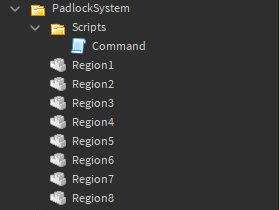# Issue with custom command

I am having issues with some code I wrote:

``````game.Players.PlayerAdded:Connect(function(plr)
plr.Chatted:Connect(function(msg,r)
local cmd = msg:split(" ")

if plr:GetRankInGroup(13843252) == RankID then

if cmd == Prefix.."toggle" then
local num = cmd
if script.Parent.Parent:FindFirstChild("Region"..num) then
local region = script.Parent.Parent["Region"..num]

if region.Transparency == 0.5 then
region.Transparency = 1
region.CanCollide = false
end

if region.Transparency == 1 then
region.Transparency = 0.5
region.CanCollide = true
end

end
end

if cmd == Prefix.."open" or Prefix.."unlock" then
local num = cmd

if script.Parent.Parent:FindFirstChild("Region"..num) then
local region = script.Parent.Parent["Region"..num]

region.Transparency = 1
region.CanCollide = false
end

end

if cmd == Prefix.."lock" or Prefix.."close" then
local num = cmd

if script.Parent.Parent:FindFirstChild("Region"..num) then
local region = script.Parent.Parent["Region"..num]

region.Transparency = 0.5
region.CanCollide = true
end

end

end
end)
end)
``````

I know it looks a bit messy but what its meant to do is open or close a barrier if a user does it.

It won’t give me any errors.

Here is the explorer by the way:Will I have to put the command script into ServerScriptService, I thought workspace and ServerScriptService would both work the same, any help is appreciated.

What is it exactly that doesn’t work?

You issue is with this and your `if cmd == Prefix.."lock" or Prefix.."close" then` statements,

The way you have them setup, the 2nd condition after the `or` will always be true because strings always give true from what I remember, thus your code will always eventually reach the close statement and make them closed

If you want to fix the the issue, you have to include `cmd ==` in the statements after the `or` too if that’s what you intended

``````if cmd == Prefix.."open" or cmd == Prefix.."unlock" then

if cmd == Prefix.."lock" or cmd == Prefix.."close" then
``````

Unless you’re not going into the code exactly, put print statements to see w here it stops

1 Like

When I enter the command nothing happens.

I will try this now, I will let you know if it works.

This still wont work, I also tried putting it in ServerScriptService, it doesnt work.

So, I did the print thing, it stops when it checks if they player is the rank in the group.

``````game.Players.PlayerAdded:Connect(function(plr)
plr.Chatted:Connect(function(msg,r)

if plr:GetRankInGroup(13843252) ~= RankID then return end

local cmd = msg:split(" ")

if cmd == Prefix.."toggle" then
local num = cmd
if script.Parent.Parent:FindFirstChild("Region"..num) then
local region = script.Parent.Parent["Region"..num]

if region.Transparency == 0.5 then
region.Transparency = 1
region.CanCollide = false
elseif region.Transparency == 1 then
region.Transparency = 0.5
region.CanCollide = true
end

end
elseif cmd == Prefix.."open" or cmd == Prefix.."unlock" then
local num = cmd

if script.Parent.Parent:FindFirstChild("Region"..num) then
local region = script.Parent.Parent["Region"..num]

region.Transparency = 1
region.CanCollide = false
end

elseif cmd == Prefix.."lock" or cmd == Prefix.."close" then
local num = cmd

if script.Parent.Parent:FindFirstChild("Region"..num) then
local region = script.Parent.Parent["Region"..num]

region.Transparency = 0.5
region.CanCollide = true
end

end
end)
end)
``````

Issues: the thingy posted by Embat, and this (above is fixed)

``````if region.Transparency == 0.5 then
region.Transparency = 1
region.CanCollide = false
end
if region.Transparency == 1 then
region.Transparency = 0.5
region.CanCollide = true
end
``````

It’s just going to set Transparency to 1 and then check if its one and change it to 0.5 lol
And yeah the reason nothing happened is there’s something off with the group rank check.
If you comment out the check it’s going to work.

Oh right, I accidentally forgot to do elseif

The group rank check was == instead of >= it was just a typo, but thank you for your solution, I probably would have never thought of the if statement.

1 Like